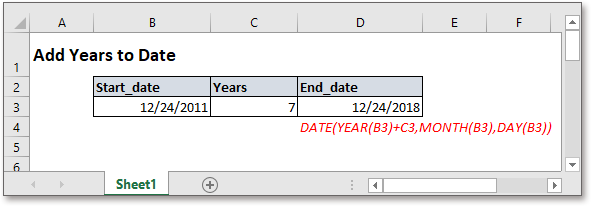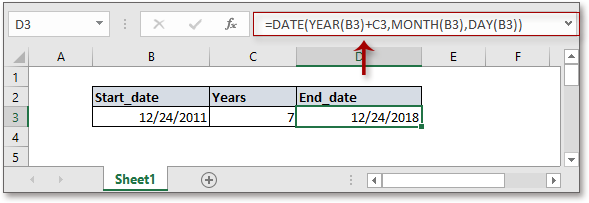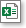## Excel Formula: Add years to dateIn some cases, you may want to add a specific number of years to a date, but it cannot directly add number of years to a given date like adding days, such as, "2020/4/1"+4 returns 2020/4/5. Here in this tutorial, it provides a formula to quickly add years to date in Excel.

Generic formula:

 DATE(YEAR(date)+years,MONTH(date),DAY(date))

Arguments

 Date: the date you used to add years. Years: a number that represents the number of years you want to add to the given date. It can be positive or negative, the formula subtracts years while the argument years is negative.

Return value

Return a value in date format.

How this formula work

Here takes an instance to explain how the formula works in Excel. Adding number of years which is placed in cell C3 to the date in cell B3, please use below formula:

 =DATE(YEAR(B3)+C3,MONTH(B3),DAY(B3))

Press Enter key to get the end date.Explanation

YEAR, MONTH and DAY functions separately get the year, month and day as relative integers from the given date.

Date function is used to create a date based on the given year, month, and day.

Sample FileClick to download sample file